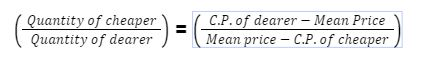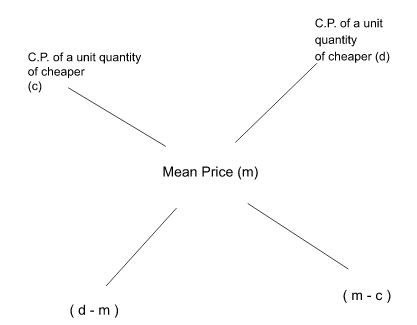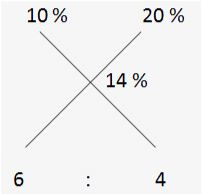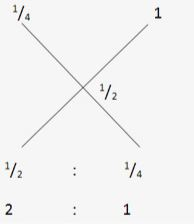Mixture and Alligation Formula For Bank Exams: Mixture and alligation formula pdf for bank exams is added in this mixture and alligation formula for bank exams article. Candidates who are sincerely preparing for their upcoming bank exams and other competitive exams shall download this mixture and alligation formula pdf for bank exams in this mixture and alligation formula for bank exams post. mixture and alligation is one of the most common topics in the quantitative aptitude section of the bank exams. In most of the bank exams like sbi clerk, sbi po, ibps po, ibps clerk, rrb po, rrb clerk and other insurance exams mixture and alligation plays a major role in the aptitude section. The mixture and alligation formula for bank exams will help you to make your calculations more easier while solving the mixture and alligation questions by using the mixture and alligation formula for bank exams. The aspirants shall download the mixture and alligation formula pdf for bank exams to maximise their scores in the bank exams.

In this mixture and alligation formula for bank exams article we have explained briefly about the definition of mixture and alligation, various mixture and alligation formula for bank exams, examples of mixture and alligation by using the mixture and alligation formula for bank exams. The aspirants who want to score maximum marks in the bank exams with speed and accuracy can download the mixture and alligation formula pdf for bank exams which is attached in this mixture and alligation formula for bank exams post.

## Mixture and Alligation Formula PDF For Bank Exams

Here we have attached the mixture and alligation formula pdf for bank exams in this  mixture and alligation formula for bank exams article. Candidates can utilize this  mixture and alligation formula for bank exams by downloading the mixture and alligation formula pdf for bank exams to make your calculations easier and for your future reference.

 More Quantitative Aptitude Formula PDFs Percentage Average Probability Time Distance Speed Time and Work Permutation and Combination Boats and Streams Simple Interest and Compound Interest Number System Mixture and Alligation Ratio and Proportion Pipes and Cisterns Sequence and Series Problems on Trains Partnership Profit and Loss

## Mixture and Alligation Formula For Bank Exams

Here we are come up with the mixture and alligation formula for bank exams. The aspirants who are getting ready for their future bank exams and other competitive exams shall exploit this mixture and alligation formula pdf for bank exams in this  mixture and alligation formula for bank exams post to solve different types of questions by using the mixture and alligation formula for bank exams.

• Alligation:

Alligation is the rule to find the ratio in which two or more ingredients at the given price must be mixed to produce a mixture of desired price.

• Mean Price:

Mean Price is the cost of a unit quantity of the mixture.

• Rule of Alligation:

If two ingredients are mixed, then(Cheaper quantity) : (Dearer quantity) = (d - m) : (m - c).

• In case a container contains x of liquid from which y units are taken out and it was replaced by water.

After n operations, the quantity of pure liquid = x ( yx )n units.

## Examples of Mixture and Alligation By Using Mixture and Alligation Formula For Bank Exams

Here we have uploaded some solve examples of mixture and alligation questions by using the mixture and alligation formula for bank exams.Aspirants can make use of this examples of mixture and alligation questions and practice more number of questions by using the mixture and alligation formula for bank exams.

Example 1:  A person has 6 litres of milk solution A which has 10 percent water in it and n litres of milk solution B which has 20 percent water in it. How many liters of solution B must he mix with solution A to obtain a milk solution 14 percent water

Solution: : Let us solve this question by both the methods i.e. the equation as well as alligation.

Here, n represent the number of litres of the 20 % solution. The amount of water in this 20 % solution [0.2n] plus the amount of water in the 10 % solution [(0.1) (6)] must be equal to the amount of water in the 14% mixture [0.14 (n + 6)].

Therefore, 0.2n + 0.1(6) = 0.14(n + 6) ⇒ 0.2n + 0.6

= 0.14n + 0.84  0.06n

=0.24 ⇒ n = 4 liters.

4 liters of the 20% salt solution must be added to the 10% solution to obtain 14% solution. This question was solved with the help of equations.

Now let us solve this question by alligation ruleExample 2:  A tank has a mixture of milk and water in the ratio of 3: 1. What proportion of the mixture should be withdrawn and replaced with water so that the resulting mixture has equal proportions of milk and water?

Solution:

Water is initially 1/4th of the total and what you are mixing in it is the water.

Water has how much water in it (seems odd). But true, water has 100 % water in it (in percentage) or 1 time of water (in fractions). So the rule of alligation will be applied as,Now they are to mixed in the ratio of 2 : 1.

It is also known that the proportion of water is actually by replacing the mixture, this means 1 out of a total of 3 (1 + 2) should be replaced i.e. 1/3rd of the total should be replaced.

 Reasoning Free Study Materials PDF Syllogism Blood Relation Coding Decoding Inequality Seating Arrangement Alphanumeric Series Number Sequence Order and Ranking Puzzle Direction Sense Floor Based Puzzle Linear Seating Arrangement Box Based Puzzle Floor and Flat Based Puzzle Year Based Puzzle Day based puzzle Age Based Puzzle Box with Stack Puzzle Parallel Seating Arrangement
 Quantitative Aptitude Free Study Materials PDF Quadratic Equation Simplification Data Interpretation Time and Work Number Series Time Distance Speed Mixture and Alligations Pipes and Cisterns Average Simple and Compound Interest Boats and Streams Problems On Trains Ages Partnership Profit Loss Discount Percentage Bar Graph DI Pie Chart DI Ratio and Proportion Radar Graph DI
 English Free Study Materials PDF Reading Comprehension Word Rearrangement Cloze Test Word Swap Sentence Rearrangement Match the column Word Usage Error Spotting Starters Sentence Improvementnt Misspelt Jumbled Sentence Pairs of Words Single Fillers Double Fillers

## Mixture and Alligation Formula For Bank Exams FAQs

Q. What is the rule of alligation?

A. Rule of Alligation:

If two ingredients are mixed, then

Quantity of cheaperQuantity of dearer = C.P. of dearer - Mean PriceMean price -     C.P. of cheaper

Q. What is alligation?

A. Alligation is the rule to find the ratio in which two or more ingredients at the             given price must be mixed to produce a mixture of desired price.

• TAGS

Dec 11 2023

Dec 11 2023

## Easiest Competitive Exams in India 2023: Top 10 Exams To Crack Easily, Bank, SSC, Railway

Dec 11 2023

###### General Awareness Smart Analysis
• Get Weekly 4 set Test
• Each Set consist of 50 Questions
• Compare your progress with Test 1 & 2 & Test 3 & 4
• Deep Analysis in topic wise questions
₹599 ₹99
###### Super Plan
• Bundle PDF Course 2023
• Ultimate Bundle PDF Course 2022
• Grand Bundle PDF Course 2021
• English Bundle PDF Course
• Insurance & Financial Market Awareness Bundle PDF  Course
• Descriptive Papers for Bank & Insurance Exams
• Interview Bundle PDF Course
• General Awareness Smart Analysis
• All Bank Exams Video Course
• All Mock Test Series (Platinum Package)
₹17990 ₹1999
###### Premium PDF Course 12 Months
• Bundle PDF Course 2024
• Prime PDF Course 2023
• Ultimate Bundle PDF Course 2022
₹5999 ₹1199
###### PRIME Bundle PDF Course 2023 (Daily Target PDF)
• Exactly Based on Real Exam Pattern
• Language: English and Hindi
• Included with All New Pattern Questions
• Covered All Major Bank (Pre + Mains Exams)
• Answer key with video solution and Quizzes
• Including Previous Year Memory based Questions
• Prepared by Top Expert Faculties
• Total 1,20,000+ Questions
• 12 Months Validity
₹9999 ₹999